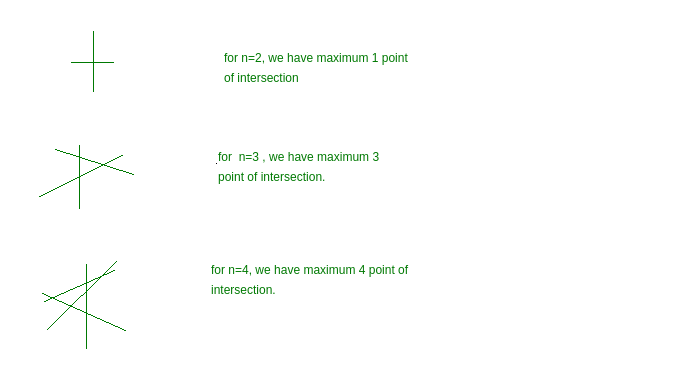# Maximum points of intersection n lines

You are given n straight lines. You have to find maximum number of point of intersection with these n lines.

Examples:

```Input : n = 4
Output : 6

Input : n = 2
Output :1
```Approach :

As we have n number of line, and we have to find maximum point of intersection using these n line. So this can be done using combination. This problem can be think as number of ways to select any two line among n line. As every line intersect with other that is selected.
So, total number of points = nC2

Below is the implementation of above approach:

## C++

 `// CPP program to find maximum intersecting ` `// points ` `#include ` `using` `namespace` `std; ` `#define ll long int ` ` `  ` `  `// nC2 = (n)*(n-1)/2; ` `ll countMaxIntersect(ll n) ` `{ ` `   ``return` `(n) * (n - 1) / 2; ` `} ` ` `  `// Driver code ` `int` `main() ` `{ ` `    ``// n is number of line ` `    ``ll n = 8; ` `    ``cout << countMaxIntersect(n) << endl; ` `    ``return` `0; ` `} `

## Java

 `// Java program to find maximum intersecting ` `// points ` ` `  `public` `class` `GFG { ` `     `  `    ``// nC2 = (n)*(n-1)/2; ` `    ``static` `long` `countMaxIntersect(``long` `n) ` `    ``{ ` `       ``return` `(n) * (n - ``1``) / ``2``; ` `    ``} ` ` `  `     `  `    ``// Driver code ` `    ``public` `static` `void` `main(String args[]) ` `    ``{ ` `        ``// n is number of line ` `        ``long` `n = ``8``; ` `        ``System.out.println(countMaxIntersect(n)); ` ` `  ` `  `    ``} ` `    ``// This code is contributed by ANKITRAI1 ` `} `

## Python3

 `# Python3 program to find maximum  ` `# intersecting points ` ` `  `#nC2 = (n)*(n-1)/2 ` `def` `countMaxIntersect(n): ` `    ``return` `int``(n``*``(n ``-` `1``)``/``2``) ` ` `  `#Driver code ` `if` `__name__``=``=``'__main__'``: ` `     `  `# n is number of line ` `    ``n ``=` `8` `    ``print``(countMaxIntersect(n)) ` ` `  `# this code is contributed by  ` `# Shashank_Sharma `

## C#

 `// C# program to find maximum intersecting ` `// points ` `using` `System; ` ` `  `class` `GFG  ` `{ ` `     `  `    ``// nC2 = (n)*(n-1)/2; ` `    ``public` `static` `long` `countMaxIntersect(``long` `n) ` `    ``{ ` `    ``return` `(n) * (n - 1) / 2; ` `    ``} ` ` `  `     `  `    ``// Driver code ` `    ``public` `static` `void` `Main() ` `    ``{ ` `        ``// n is number of line ` `        ``long` `n = 8; ` `        ``Console.WriteLine(countMaxIntersect(n)); ` `    ``} ` `} ` `// This code is contributed by Soumik `

## PHP

 ` `

Output:

```28
```

Attention reader! Don’t stop learning now. Get hold of all the important DSA concepts with the DSA Self Paced Course at a student-friendly price and become industry ready.

My Personal Notes arrow_drop_upCheck out this Author's contributed articles.

If you like GeeksforGeeks and would like to contribute, you can also write an article using contribute.geeksforgeeks.org or mail your article to contribute@geeksforgeeks.org. See your article appearing on the GeeksforGeeks main page and help other Geeks.

Please Improve this article if you find anything incorrect by clicking on the "Improve Article" button below.